## 9.8 Exercises

Exercise 9.1:
Students have to make decisions about how much to study for each course. The aim of this question is to investigate how to use decision networks to help them make such decisions.

Suppose students first have to decide how much to study for the midterm. They can study a lot, study a little, or not study at all. Whether they pass the midterm depends on how much they study and on the difficulty of the course. As a first-order approximation, they pass if they study hard or if the course is easy and they study a bit. After receiving their midterm grade, they have to decide how much to study for the final exam. Again, the final exam result depends on how much they study and on the difficulty of the course. Their final grade depends on which exams they pass; generally they get an A if they pass both exams, a B if they only pass the final, a C if they only pass the midterm, or an F if they fail both. Of course, there is a great deal of noise in these general estimates.

Suppose that their final utility depends on their total effort and their final grade. Suppose the total effort is obtained by adding the effort in studying for the midterm and the final.

1. Draw a decision network for a student decision based on the preceding story.
2. What is the domain of each variable?
3. Give appropriate conditional probability tables.
4. What is the best outcome (give this a utility of 100) and what is the worst outcome (give this a utility of 0)?
5. Give an appropriate utility function for a student who just wants to pass (not get an F). What is an optimal policy for this student?
6. Give an appropriate utility function for a student who wants to do really well. What is an optimal policy for this student?
Exercise 9.2:
Consider the following decision network: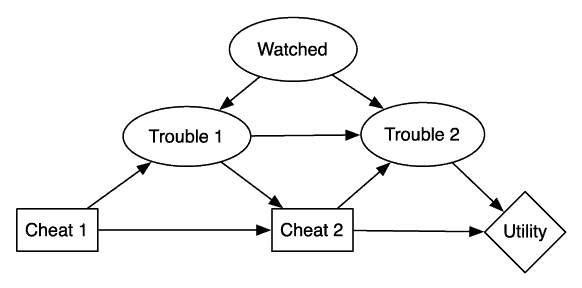This diagram models a decision about whether to cheat at two different time instances.

Suppose P(watched)=0.4, P(trouble1|cheat1,watched)=0.8, and Trouble1 is true with probability 0 for the other cases. Suppose the conditional probability P(Trouble2|Cheat2,Trouble1,Watched) is given by the following table:

 Cheat2 Trouble1 Watched P(Trouble2=t) t t t 1.0 t t f 0.3 t f t 0.8 t f f 0.0 f t t 0.3 f t f 0.3 f f t 0.0 f f f 0.0

Suppose the utility is given by

 Trouble2 Cheat2 Utility t t 30 t f 0 f t 100 f f 70
1. What is an optimal decision function for the variable Cheat2? Show what factors are created. Please try to do it by hand, and then check it with the AISpace.org applet.
2. What is an optimal policy? What is the value of an optimal policy? Show the tables created.
3. What is an optimal policy if the probability of being watched goes up?
4. What is an optimal policy when the rewards for cheating are reduced?
5. What is an optimal policy when the instructor is less forgiving (or less forgetful) of previous cheating?
Exercise 9.3:
Suppose that, in a decision network, the decision variable Run has parents Look and See. Suppose you are using VE to find an optimal policy and, after eliminating all of the other variables, you are left with the factor
 Look See Run Value true true yes 23 true true no 8 true false yes 37 true false no 56 false true yes 28 false true no 12 false false yes 18 false false no 22
1. What is the resulting factor after eliminating Run? [Hint: You do not sum out Run because it is a decision variable.]
2. What is the optimal decision function for Run?
Exercise 9.4:
Suppose that, in a decision network, there were arcs from random variables "contaminated specimen" and "positive test" to the decision variable "discard sample." Sally solved the decision network and discovered that there was a unique optimal policy:
 contaminated specimen positive test discard sample true true yes true false no false true yes false false no

What can you say about the value of information in this case?

Exercise 9.5:
How sensitive are the answers from the decision network of Example 9.13 to the probabilities? Test the program with different conditional probabilities and see what effect this has on the answers produced. Discuss the sensitivity both to the optimal policy and to the expected value of the optimal policy.
Exercise 9.6:
In Example 9.13, suppose that the fire sensor was noisy in that it had a 20% false-positive rate,
P(see_smoke | report ∧¬smoke)=0.2,

and a 15% false negative-rate:

P(see_smoke | report ∧smoke)=0.85.

Is it still worthwhile to check for smoke?

Exercise 9.7:
Consider the belief network of Exercise 6.8. When an alarm is observed, a decision is made whether to shut down the reactor. Shutting down the reactor has a cost cs associated with it (independent of whether the core was overheating), whereas not shutting down an overheated core incurs a cost cm that is much higher than cs.
1. Draw the decision network to model this decision problem for the original system (i.e., with only one sensor).
2. Specify the tables for all new factors that must be defined (you should use the parameters cs and cm where appropriate in the tables). Assume that the utility is the negative of cost.
Exercise 9.8:
Explain why we often use discounting of future rewards in MDPs. How would an agent act differently if the discount factor was 0.6 as opposed to 0.9?
Exercise 9.9:
Consider a game world: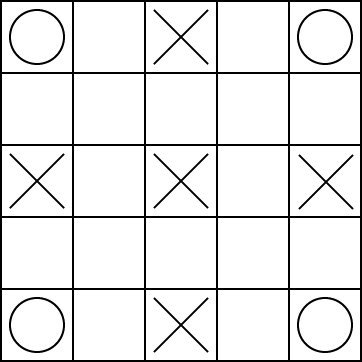The robot can be at one of the 25 locations on the grid. There can be a treasure on one of the circles at the corners. When the robot reaches the corner where the treasure is, it collects a reward of 10, and the treasure disappears. When there is no treasure, at each time step, there is a probability P1=0.2 that a treasure appears, and it appears with equal probability at each corner. The robot knows its position and the location of the treasure.

There are monsters at the squares marked with an X. Each monster randomly and independently, at each time step, checks if the robot is on its square. If the robot is on the square when the monster checks, it has a reward of -10 (i.e., it loses 10 points). At the center point, the monster checks at each time step with probability p2=0.4; at the other 4 squares marked with an X, the monsters check at each time step with probability p3=0.2.

Assume that the rewards are immediate upon entering a state: that is, if the robot enters a state with a monster, it gets the (negative) reward on entering the state, and if the robot enters the state with a treasure, it gets the reward upon entering the state, even if the treasure arrives at the same time.

The robot has 8 actions corresponding to the 8 neighboring squares. The diagonal moves are noisy; there is a p4=0.6 probability of going in the direction chosen and an equal chance of going to each of the four neighboring squares closest to the desired direction. The vertical and horizontal moves are also noisy; there is a p5=0.8 chance of going in the requested direction and an equal chance of going to one of the adjacent diagonal squares. For example, the actions up-left and up have the following result: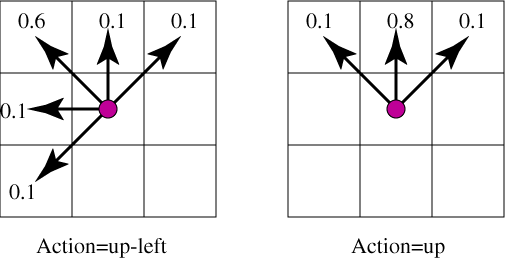If the action would result in crashing into a wall, the robot has a reward of -2 (i.e., loses 2) and does not move.

There is a discount factor of p6=0.9.

1. How many states are there? (Or how few states can you get away with?) What do they represent?
2. What is an optimal policy?
3. Suppose the game designer wants to design different instances of the game that have non-obvious optimal policies for a game player. Give three assignments to the parameters p1 to p6 with different optimal policies. If there are not that many different optimal policies, give as many as there are and explain why there are no more than that.
Exercise 9.10:
Consider a 5×5 grid game similar to the game of the previous question. The agent can be at one of the 25 locations, and there can be a treasure at one of the corners or no treasure.

In this game the "up" action has dynamics given by the following diagram: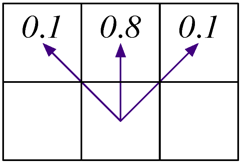That is, the agent goes up with probability 0.8 and goes up-left with probability 0.1 and up-right with probability 0.1.

If there is no treasure, a treasure can appear with probability 0.2. When it appears, it appears randomly at one of the corners, and each corner has an equal probability of treasure appearing. The treasure stays where it is until the agent lands on the square where the treasure is. When this occurs the agent gets an immediate reward of +10 and the treasure disappears in the next state transition. The agent and the treasure move simultaneously so that if the agent arrives at a square at the same time the treasure appears, it gets the reward.

Suppose we are doing asynchronous value iteration and have the value for each state as in the following grid. The numbers in the square represent the value of that state and empty squares have a value of zero. It is irrelevant to this question how these values got there.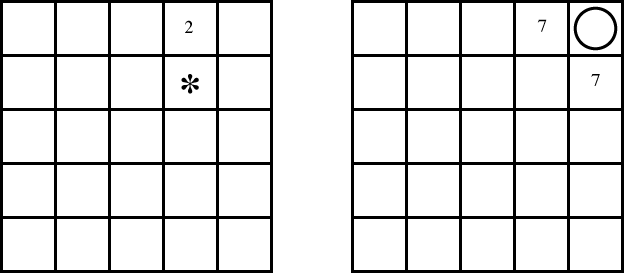The left grid shows the values for the states where there is no treasure and the right grid shows the values of the states when there is a treasure at the top-right corner. There are also states for the treasures at the other three corners, but you assume that the current values for these states are all zero.

Consider the next step of asynchronous value iteration. For state s13, which is marked by * in the figure, and the action a2, which is "up," what value is assigned to Q[s13,a2] on the next value iteration? You must show all work but do not have to do any arithmetic (i.e., leave it as an expression). Explain each term in your expression.

Exercise 9.11:
In a decision network, suppose that there are multiple utility nodes, where the values must be added. This lets us represent a generalized additive utility function. How can the VE for decision networks algorithm, shown in Figure 9.11, be altered to include such utilities?
Exercise 9.12:
How can variable elimination for decision networks, shown in Figure 9.11, be modified to include additive discounted rewards? That is, there can be multiple utility (reward) nodes, having to be added and discounted. Assume that the variables to be eliminated are eliminated from the latest time step forward.
Exercise 9.13:
This is a continuation of Exercise 6.8.
1. When an alarm is observed, a decision is made whether to shut down the reactor. Shutting down the reactor has a cost cs associated with it (independent of whether the core was overheating), whereas not shutting down an overheated core incurs a cost cm, which is much higher than cs. Draw the decision network modeling this decision problem for the original system (i.e., only one sensor). Specify any new tables that must be defined (you should use the parameters cs and cm where appropriate in the tables). You can assume that the utility is the negative of cost.
2. For the decision network in the previous part, determine the expected utility of shutting down the reactor versus not shutting it down when an alarm goes off. For each variable eliminated, show which variable is eliminated, how it is eliminated (through summing or maximization), which factors are removed, what factor is created, and what variables this factor is over. You are not required to give the tables.
Exercise 9.14:
One of the decisions we must make in real life is whether to accept an invitation even though we are not sure we can or want to go to an event. The following figure represents a decision network for such a problem: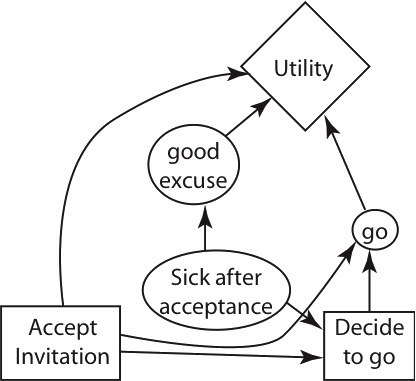Suppose that all of the decision and random variables are Boolean (i.e., have domain {true,false}). You can accept the invitation, but when the time comes, you still must decide whether or not to go. You might get sick in between accepting the invitation and having to decide to go. Even if you decide to go, if you haven't accepted the invitation you may not be able to go. If you get sick, you have a good excuse not to go. Your utility depends on whether you accept, whether you have a good excuse, and whether you actually go.

1. Give a table representing a possible utility function. Assume the unique best outcome is that you accept the invitation, you don't have a good excuse, but you do go. The unique worst outcome is that you accept the invitation, you don't have a good excuse, and you don't go. Make your other utility values reasonable.
2. Suppose that that you get to observe whether you are sick before accepting the invitation. Note that this is a different variable than if you are sick after accepting the invitation. Add to the network so that this situation can be modeled. You must not change the utility function, but the new observation must have a positive value of information. The resulting network must be one for which the decision network solving algorithm works.
3. Suppose that, after you have decided whether to accept the original invitation and before you decide to go, you can find out if you get a better invitation (to an event that clashes with the original event, so you cannot go to both). Suppose you would rather go to the better invitation than go to the original event you were invited to. (The difficult decision is whether to accept the first invitation or wait until you get a better invitation, which you may not get.) Unfortunately, having another invitation does not provide a good excuse. On the network, add the node "better invitation" and all relevant arcs to model this situation. [You do not have to include the node and arcs from part (b).]
4. If you have an arc between "better invitation" and "accept invitation" in part (c), explain why (i.e., what must the world be like to make this arc appropriate). If you did not have such an arc, which way could it go to still fit the preceding story; explain what must happen in the world to make this arc appropriate.
5. If there was no arc between "better invitation" and "accept invitation" (whether or not you drew such an arc), what must be true in the world to make this lack of arc appropriate.
Exercise 9.15:
Consider the following decision network: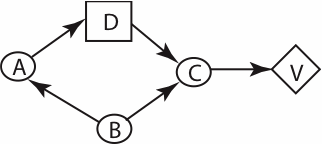1. What are the initial factors. (You do not have to give the tables; just give what variables they depend on.)
2. Show what factors are created when optimizing the decision function and computing the expected value, for one of the legal elimination orderings. At each step explain which variable is being eliminated, whether it is being summed out or maximized, what factors are being combined, and what factors are created (give the variables they depend on, not the tables).
3. If the value of information of A at decision D is zero, what does an optimal policy look like? (Please give the most specific statement you can make about any optimal policy.)
Exercise 9.16:
What is the main difference between asynchronous value iteration and standard value iteration? Why does asynchronous value iteration often work better than standard value iteration?
Exercise 9.17:
Consider a grid world where the action "up" has the following dynamics:That is, it goes up with probability 0.8, up-left with probability 0.1, and up-right with probability 0.1. Suppose we have the following states: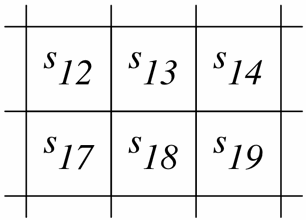There is a reward of +10 upon entering state s14, and a reward of -5 upon entering state s19. All other rewards are 0.

The discount is 0.9.

Suppose we are doing asynchronous value iteration, storing Q[S,A], and we have the following values for these states:

 V(s12)=5 V(s13)=7 V(s14)=-3 V(s17)=2 V(s18)=4 V(s19)=-6

Suppose, in the next step of asynchronous value iteration, we select state s18 and action up. What is the resulting updated value for Q[s18,up]? Give the numerical formula, but do not evaluate or simplify it.